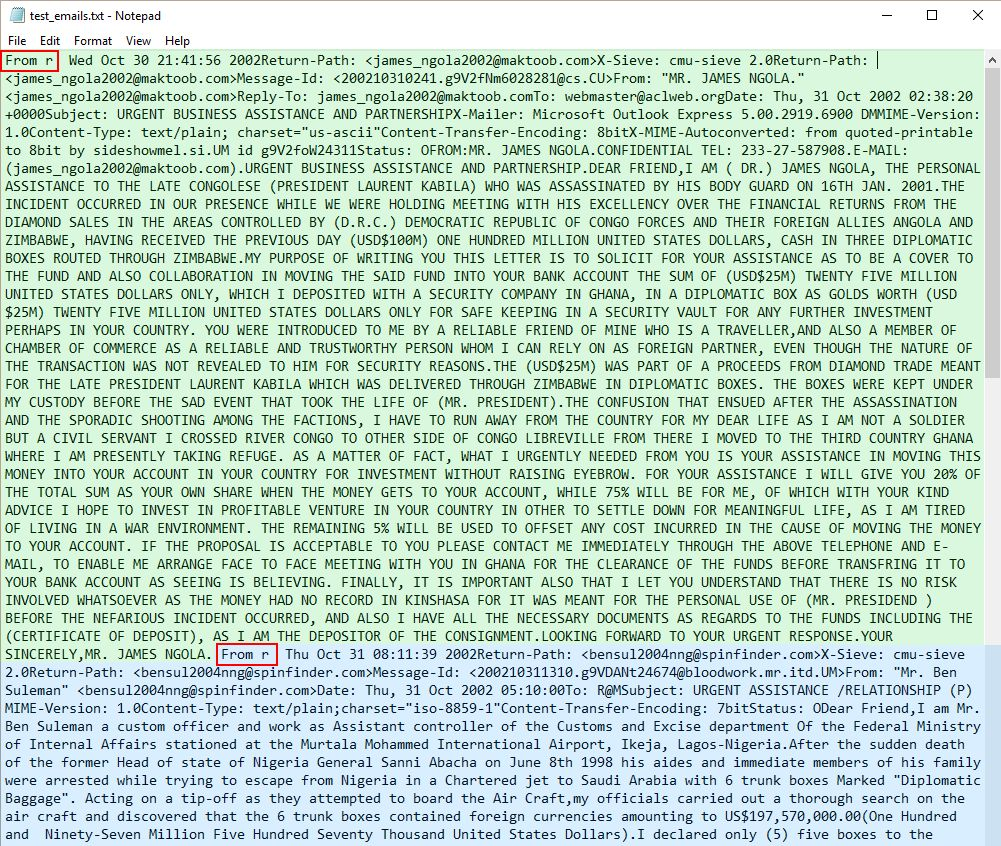### 热门观点更多## 人工智能将让无数职业过时，如何规划孩子的职业生涯

2017-12-18 10:36:56## 人工智能的黑暗秘密：如何让 AI 解释自身行为？

2017-12-15 13:23:24## 科技巨头间的人才争夺战，正从硅谷蔓延至全球

2017-09-21 20:25:08## “AT”竞相追逐互联网保险大蛋糕，谁会站在资源优势的制高点？

2017-08-15 12:39:50## 腾讯 COO 任宇昕：国内信息安全投入不足 1%

2017-08-15 12:29:04

## 数据科学入门必读：如何使用正则表达式？

2018-04-16 12:53:36 人工智能 | , ,``fh = open(r"test_emails.txt", "r").read()``

``````for line in fh.split("\n"):
if "From:" in line:
print(line)``````

``````import re

for line in re.findall("From:.*", fh):
print(line)``````

re.findall() 返回字符串中满足其模式的所有实例的列表。这是 Python 内置的 re 模块中最常用的函数之一。分解看看。该函数的形式是 re.findall(pattern, string)，有两个参数。其中，pattern 表示我们希望寻找的子字符串，string 表示我们要在其中查找的主字符串。主字符串可以包含很多行。

.* 是字符串模式的简写。我们马上就会详细解释。现在只需知道它们的作用是匹配 From: 字段中的名称和电子邮箱地址。

• \w 匹配字母数字字符，即 a-z、A-Z 和 0-9，也会匹配下划线 _ 和连接号 –
• \d 匹配数字，即 0-9
• \s 匹配空白字符，包括制表符、换行符、回车符和空格符
• \S 匹配非空白字符
• . 匹配除换行符 \n 之外的任意字符

``````for line in re.findall("From:.", fh):
print(line)``````

``````for line in re.findall("From:...........", fh):
print(line)``````

* 匹配 0 个或更多个其左侧的模式的实例。也就是说它会查找重复的模式。当我们查找重复模式时，我们说我们的搜索是「贪婪匹配」。如果我们没有查找重复模式，我们可以说我们的搜索是「非贪婪匹配」或「懒惰匹配」。

``````for line in re.findall("From:.*", fh):
print(line)``````

``````match = re.findall("From:.*", fh)

for line in match:
print(re.findall("\".*\"", line))``````

``````match = re.findall("From:.*", fh)

for line in match:
print(re.findall("\w\S*@.*\w", line))``````

``````for line in match:
print(re.findall("\w\S*@", line))``````

``````for line in match:
print(re.findall("@.*", line))``````

``````for line in match:
print(re.findall("@.*\w", line))``````

re.findall() 毫无疑问非常有用，re 模块还提供了一些同样方便的函数，其中包括：

• re.search()
• re.split()
• re.sub()

re.search()

re.findall() 匹配的是一个模式在一个字符串中的所有实例然后以列表的形式返回它们，而 re.search() 匹配的是一个模式在一个字符串中的第一个实例，然后以 re 匹配对象的形式返回它。

``````match = re.search("From:.*", fh)
print(type(match))
print(type(match.group()))
print(match)
print(match.group())``````

re.split()

``````address = re.findall("From:.*", fh)
for line in re.findall("\w\S*@.*\w", item):

re.sub()

re.sub() 是另一个很好用的 re 函数。顾名思义，它的功能是替换一个字符串的一部分。举个例子：

``````sender = re.search("From:.*", fh)
print(email)``````

re.sub() 有三个参数。第一个是所要替换的子字符串，第二个是用来替换前者的字符串，第三个是主字符串本身。

pandas 的正则表达式

• sender_name（发件人名称）
• recipient_name（收件人名称）
• date_sent（发送时间）
• subject（主题）
• email_body（邮件正文）

``````import re
import pandas as pd
import email

emails = []

``````contents = re.split(r"From r", fh)
contents.pop(0)``````「From r」起头的电子邮件

``````for item in contents:
emails_dict = {}``````

``````for item in contents: # First two lines again so that Jupyter runs the code.
emails_dict = {}

# Find sender's email address and name.

# Step 1: find the whole line beginning with "From:".
sender = re.search(r"From:.*", item)
``````

``````# Step 2: find the email address and name.
if sender is not None:
s_email = re.search(r"\w\S*@.*\w", sender.group())
s_name = re.search(r":.*<", sender.group())
else:
s_email = None
s_name = None``````

``````print("sender type: " + str(type(sender)))
print("sender.group() type: " + str(type(sender.group())))
print("sender: " + str(sender))
print("sender.group(): " + str(sender.group()))
print("\n")``````

``````print(s_email)
print(s_name)``````

``````# Step 3A: assign email address as string to a variable.
if s_email is not None:
sender_email = s_email.group()
else:
sender_email = None

emails_dict["sender_email"] = sender_email``````

``````# Step 3B: remove unwanted substrings, assign to variable.
if s_name is not None:
sender_name = re.sub("\s*<", "", re.sub(":\s*", "", s_name.group()))
else:
sender_name = None

# Add sender's name to dictionary.
emails_dict["sender_name"] = sender_name``````

``````print(sender_email)
print(sender_name)``````

``recipient = re.search(r"To:.*", item)``

``````if recipient is not None:
r_email = re.search(r"\w\S*@.*\w", recipient.group())
r_name = re.search(r":.*<", recipient.group())
else:
r_email = None
r_name = None``````

``````if r_email is not None:
recipient_email = r_email.group()
else:
recipient_email = None

emails_dict["recipient_email"] = recipient_email

if r_name is not None:
recipient_name = re.sub("\s*<", "", re.sub(":\s*", "", r_name.group()))
else:
recipient_name = None

emails_dict["recipient_name"] = recipient_name``````

``````for item in contents: # First two lines again so that Jupyter runs the code.
emails_dict = {}

date_field = re.search(r"Date:.*", item)``````

``````if date_field is not None:
date = re.search(r"\d+\s\w+\s\d+", date_field.group())
else:
date = None

print(date_field.group())``````

``````if date is not None:
date_sent = date.group()
date_star = date_star_test.group()
else:
date_sent = None

emails_dict["date_sent"] = date_sent``````

``````date = re.search(r"\d+\s\w+\s\d+", date_field.group())

# What happens when we use * instead?
date_star_test = re.search(r"\d*\s\w*\s\d*", date_field.group())

date_sent = date.group()
date_star = date_star_test.group()

print(date_sent)
print(date_star)``````

``````for item in contents: # First two lines again so that Jupyter runs the code.
emails_dict = {}

subject_field = re.search(r"Subject: .*", item)

if subject_field is not None:
subject = re.sub(r"Subject: ", "", subject_field.group())
else:
subject = None

emails_dict["subject"] = subject
``````

``````full_email = email.message_from_string(item)
emails_dict["email_body"] = body``````

[ ] 匹配放置于其中的任意字符。比如如果我们想在一个字符串中查找 a、b 或 c，我们可以使用 [abc] 作为模式。我们前面讨论的模式也适用。[\w\s] 是查找字母数字或空白字符。但 . 是例外，它在 [ ] 中就表示句号。

``emails.append(emails_dict)``

``````import re
import pandas as pd
import email

emails = []

contents = re.split(r"From r",fh)
contents.pop(0)

for item in contents:
emails_dict = {}

sender = re.search(r"From:.*", item)

if sender is not None:
s_email = re.search(r"\w\S*@.*\w", sender.group())
s_name = re.search(r":.*<", sender.group())
else:
s_email = None
s_name = None

if s_email is not None:
sender_email = s_email.group()
else:
sender_email = None

emails_dict["sender_email"] = sender_email

if s_name is not None:
sender_name = re.sub("\s*<", "", re.sub(":\s*", "", s_name.group()))
else:
sender_name = None

emails_dict["sender_name"] = sender_name

recipient = re.search(r"To:.*", item)

if recipient is not None:
r_email = re.search(r"\w\S*@.*\w", recipient.group())
r_name = re.search(r":.*<", recipient.group())
else:
r_email = None
r_name = None

if r_email is not None:
recipient_email = r_email.group()
else:
recipient_email = None

emails_dict["recipient_email"] = recipient_email

if r_name is not None:
recipient_name = re.sub("\s*<", "", re.sub(":\s*", "", r_name.group()))
else:
recipient_name = None

emails_dict["recipient_name"] = recipient_name

date_field = re.search(r"Date:.*", item)

if date_field is not None:
date = re.search(r"\d+\s\w+\s\d+", date_field.group())
else:
date = None

if date is not None:
date_sent = date.group()
else:
date_sent = None

emails_dict["date_sent"] = date_sent

subject_field = re.search(r"Subject: .*", item)

if subject_field is not None:
subject = re.sub(r"Subject: ", "", subject_field.group())
else:
subject = None

emails_dict["subject"] = subject

# "item" substituted with "email content here" so full email not displayed.

full_email = email.message_from_string(item)
emails_dict["email_body"] = "email body here"

emails.append(emails_dict)

# Print number of dictionaries, and hence, emails, in the list.
print("Number of emails: " + str(len(emails_dict)))

print("\n")

# Print first item in the emails list to see how it looks.
for key, value in emails.items():
print(str(key) + ": " + str(emails[key]))``````

``````import pandas as pd # Module imported above, imported again as reminder.
emails_df = pd.DataFrame(emails)``````

``pd.DataFrame.head(emails_df, n=3)``

dataframe.head() 函数只会展示前面几行，而不会展示整个数据集。它有一个参数。还有一个可选参数可以指定所要展示的行数。这里 n=3 表示我们想看 3 行。

| 看起来似乎和 [ ] 一样，但实际并不一样。假如我们要查找 crab 或 lobster 或 isopod，那么使用 crab|lobster|isopod 会比使用 [crablobsterisopod] 要合理得多。前者是查找其中每个词，而后者是搜索其中每个字母。

``emails_df[emails_df["sender_email"].str.contains("maktoob|spinfinder")]``

emails_df[‘sender_email’] 是选择有 sender_email 标签的一列。接下来，str.contains(maktoob|spinfinder) 是指如果在该列中找到了 maktoob 或 spinfinder，则返回 Ture。最后，外围的 emails_df[] 返回一个行视图，其中 sender_email 列包含了目标子字符串。干的漂亮！

``````# Step 1: find the index where the "sender_email" column contains "@maktoob.com".
index = emails_df[emails_df["sender_email"].str.contains(r"\w\S*@maktoob.com")].index.values``````

``````# Step 2: use the index to find the value of the cell in the "sender_email" column.
# The result is returned as pandas Series object

``````# Step 3: extract the email address, which is at index 0 in the Series object.

``````# Step 4: find the value of the "email_body" column where the "sender email" column is address_string.IT思维（itsiwei.com）是互联网首个定位在科技与电商“思维”韬略的平台，我们时刻关注互联网电商行业新动向； 诚邀行业资深从业者加入“思维客家族”！Return to Top ▲